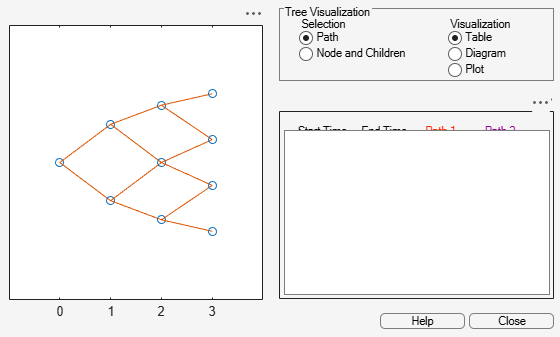treepath

Entries from node of recombining binomial tree

Description

example

Values = treepath(Tree,BranchList) extracts entries of a node of a recombining binomial tree. The node path is described by the sequence of branchings taken, starting at the root. The top branch is number one, the second-to-top is two, and so on. Set the branch sequence to zero to obtain the entries at the root node.

Examples

collapse all

FwdRates = treepath(BDTTree.FwdTree, [1 2 1])
FwdRates = 4×1

1.1000
1.0979
1.1377
1.1183

This returns the rates at the tree nodes located by taking the up branch, then the down branch, and finally the up branch again.

You can visualize this with the treeviewer function.

treeviewer(BDTTree)Input Arguments

collapse all

Recombining binomial tree or trinomial tree specified as a struct that is created using one of the following functions:

Data Types: struct

Number of paths (NUMPATHS) by path length (PATHLENGTH), specified as a matrix containing the sequence of branchings.

Data Types: double

Output Arguments

collapse all

Retrieved entries of a recombining tree, returned a number of values (NUMVALS)-by-NUMPATHS matrix.

Introduced before R2006a